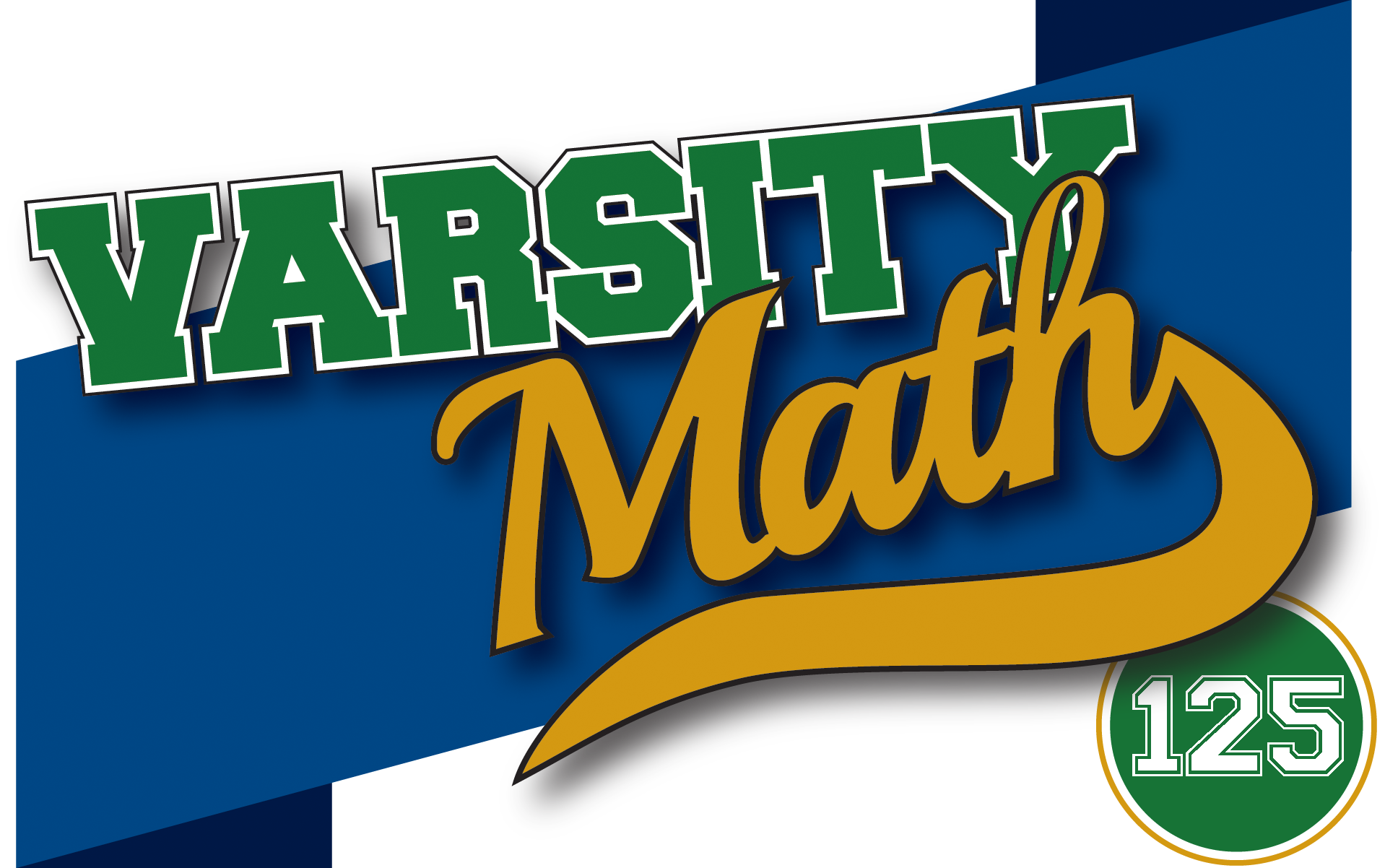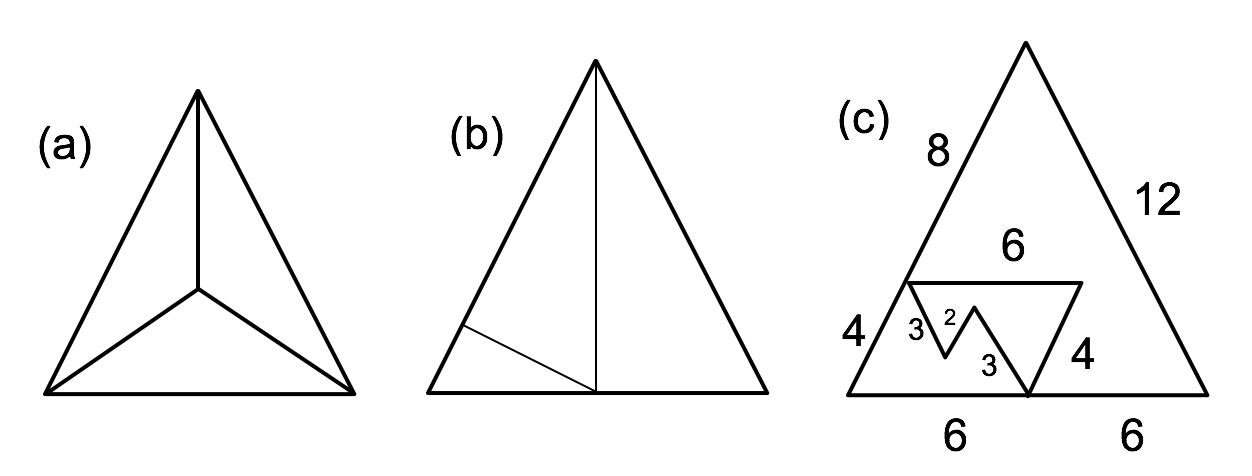## ________________

The coach provides puzzles involving digits as today’s fare.

## ________________### Piece of Pi

The coach notes that somewhere in the digits of pi, the entirety of any text is encoded if the following scheme is used: space = 00, A = 01, a = 02, B = 03, b = 04 and so on. If we assume random digits in the expansion of pi, it will contain the expression “National Museum of Mathematics” in consecutive order if enough digits are examined.

About how many digits of pi will have to be examined to get a 50% probability of encountering a string of 60 consecutive decimal digits that encodes the museum’s name according to the coach’s scheme?

### Making 17 and 27

Produce math expressions in which you may use only +, -, ×, ÷, exponents, decimal points, and parentheses.

(a) Make 17 using 1, 4, and 5 one time each.
(b) Make 27 using 1, 5, and 9 one time each.

## Solutions to week 124

The figures below show the three solutions to Cutting the Triangle, and the solution to Square Repair. Note that Puzzle (a) of Cutting the Triangle has an infinite number of additional solutions.

Cutting the TriangleSquare Repair## Recent Weeks

Links to all of the puzzles and solutions are on the Complete Varsity Math page.

Come back next week for answers and more puzzles.